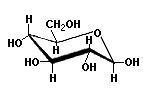# How can I draw d -glucose in its chair conformation? Why it is the most common aldohexose in nature?

Jun 15, 2015

First convert the Fischer projection to a Haworth projection, then convert the Haworth projection to a chair form.

#### Explanation:

The Fischer projection of glucose isConvert to a Haworth Projection

Step 1. Draw a basic Haworth projection with the ring oxygen at the top.$\text{C-1}$ is the atom to the right of the oxygen, and $\text{C-5}$ is the atom to its left.

Step 2. Draw a $\text{CH"_2"OH}$ on $\text{C-5}$.Step 3. Draw an $\text{OH}$ below the ring on $\text{C-1}$ for the α form (draw it above the ring for the β form).Step 4. Draw all the $\text{OH}$ groups on the right side of the Fischer projection on the bottom of the ring. Those on the left go above the ring.

The $\text{O}$ on C-5 is part of the ring.You can omit the hydrogen atoms, so the Haworth projection for α-D-glucopyranose isConvert Haworth to Chair

Step 1. Draw a cyclohexane chair in which the $\text{O}$ atom replaces $\text{C-6}$ and the bulky $\text{CH"_2"OH}$ is in the equatorial position.Step 2. Put all the $\text{OH}$ groups that are "down" in the Haworth projection "down" in the chair. All the other $\text{OH}$ groups go "up".

The chair form of α-D-glucopyranose isThe structure of β-D-glucopyranose isPrevalence of Glucose

As you move around the β-glucose ring, you see that all the substituents are equatorial.

This is the most stable arrangement possible.

In α-glucose, only the $\text{OH}$ at $\text{C-1}$ is axial.

Every other aldohexose would have more axial substituents and be less stable.

Glucose is the most common hexose because it is the most stable.